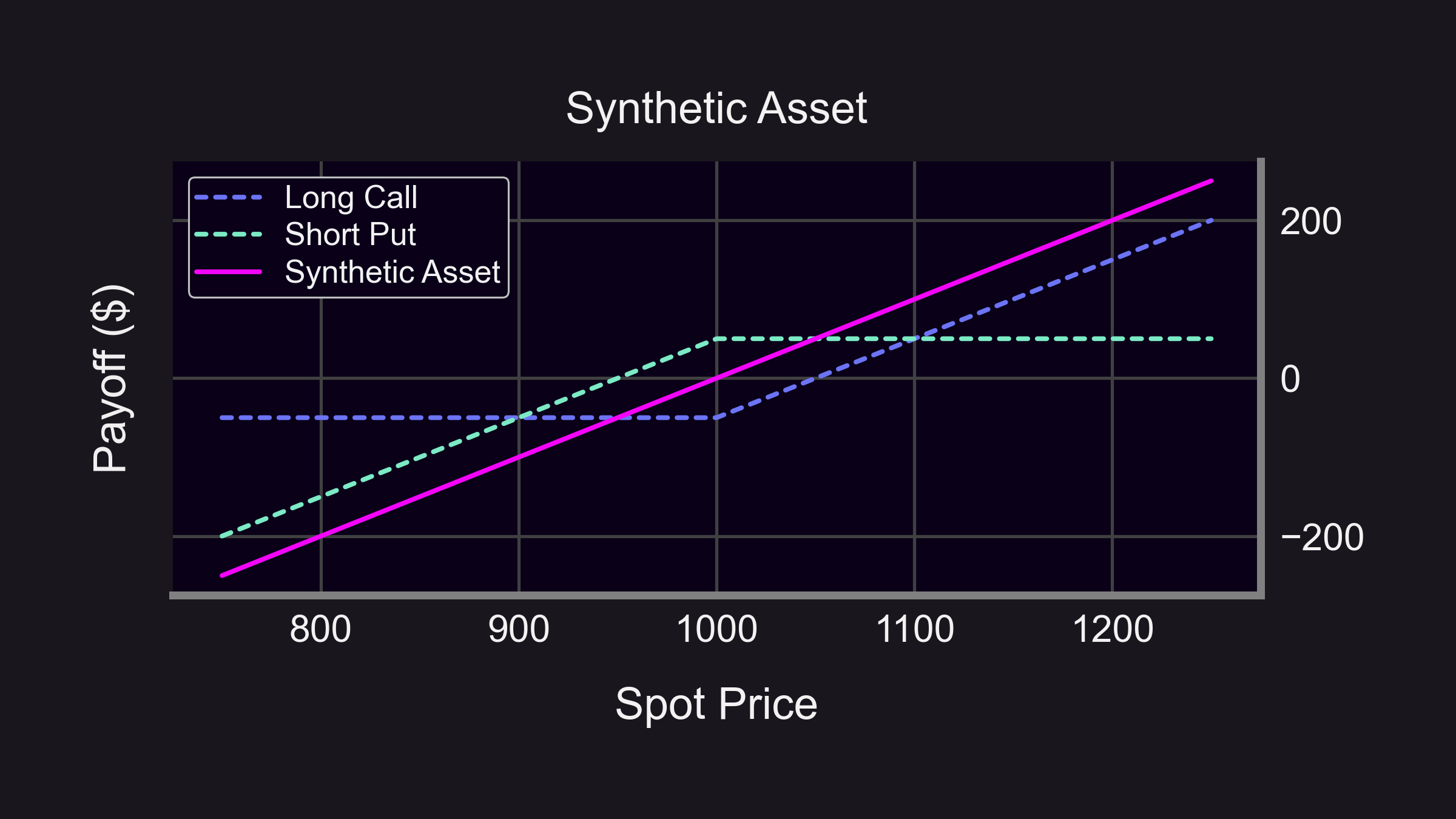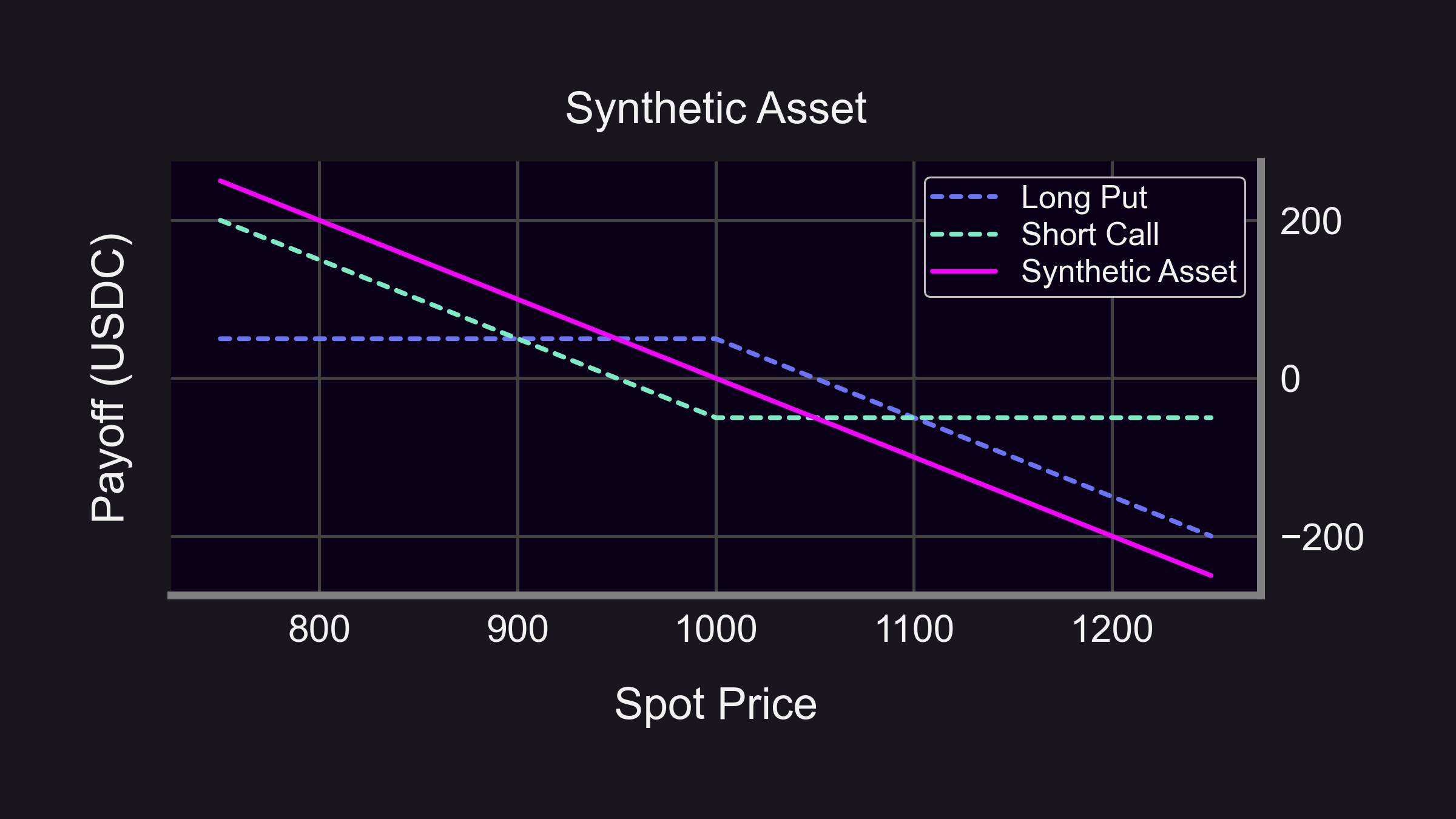Advanced options trading strategies are designed to help traders achieve their financial objectives while minimizing their risk exposure.

1. Defined/undefined risk
3. Synthetic positions

## Managing Defined/Undefined Risk​

Options strategies can be divided into two types of risks:

1. Defined risk: Strategy where there is a maximum possible loss which is known in advance.
2. Undefined risk: Strategy where there is potential for unlimited loss.

Managing risk is crucial when trading options, and it's important to understand how to do so effectively.

### Defined Risk Options Trading Strategy​

A popular defined risk options trading strategy is a bull call spread. This strategy involves buying a call option at a lower strike price and selling a call option at a higher strike price.

This strategy limits both the potential profit and potential loss of the trade.

If ETH price rises above the strike price, e.g. $1,200, the call option can be exercised, and you will be obligated to sell 1 ETH at$1,000. But because you do not own any ETH (this is a naked position), you must acquire ETH on the open market by buying ETH at the current price ($1,200). Thus, you purchase ETH for a high price ($1,200) and sell it at a low price ($1,000) for a loss ($125 + $1,000 -$1,200 = -$75). However, if the option is not exercised and ETH price continues to rise, your losses would increase (without limit). ## Spreads​ A spread is an options trading strategy that involves buying and selling options (each option is a "leg") on the same underlying asset. However, the strike price or expiration date (in TradFi) of each leg may differ. Spreads can be used to limit risk and increase potential profit. ### Vertical Spread​ One type of spread is a vertical spread. A vertical spread involves buying and selling options with the same expiration date (in TradFi) but different strike prices. For example, if you're bullish on ETH, you can buy a call option with a lower strike price and sell a call option with a higher strike price. This strategy will limit your risk while giving you the potential for profit if ETH price stays between the two strikes. This is an example of a bull call spread, which is also described above. ### Horizontal Spread​ Another type of spread is the horizontal spread (also called a calendar spread). A horizontal spread involves buying and selling options with the same strike price but different expiration dates. This strategy can be used to profit from changes in the implied volatility of the options. ### Diagonal Spread​ Another type of spread is the diagonal spread (also called the "Poor Man's Covered Call"). A diagonal spread involves buying and selling options with different strike prices and different expiration dates. While its payoff is similar to that of a covered call, its capital requirements are much lower. This makes the diagonal spread a capital-efficient version of the covered call. ## Synthetic Positions​ A synthetic position is a trading strategy that involves creating a position that mimics the risk/reward profile of another position. Typically, this results in higher capital efficiency, as you are exposed to the profit profiles of some position without actually holding it. ### Synthetic Long Position​ A synthetic long position is a trading strategy that uses options to mimic the risk/reward profile of owning some asset. To create a synthetic long position, a trader can buy a call option and sell a put option with the same strike price and the same expiration date (in TradFi).For example, let's say a trader wants to create a synthetic long position on ETH, which is currently trading at$100. The trader can buy a call option with a $100 strike price and sell a put option with a$100 strike price, both expiring in one month (in TradFi).

At the end of one month (in TradFi options), the profit from the synthetic position is similar to the profit of purchasing ETH outright:

1. If ETH price rises above $100, e.g. to$120, the trader's long call option is ITM with intrinsic value of $20, while the trader's short put option expires OTM. The intrinsic value is the same as the profit from purchasing ETH outright ($20).

2. If ETH price falls below $100, e.g. to$90, the trader's long call option expires worthless, while the trader's short put option is ITM with intrinsic value of $10. The intrinsic value (which is a loss to the trader who sold the put) is the same as the loss from purchasing ETH outright ($10).

3. If ETH price remains at $100, both options expire worthless with intrinsic values of$0. This is the same as the PnL from purchasing ETH outright ($0). In every case, the synthetic position's value is similar to buying the asset outright. However, there are also some differences: • In the synthetic position, the trader had to purchase the call option (e.g. for a$7 premium) and sell the put option (e.g. for a $5 premium). This is a total cost of$2 ($7 -$5).
• The trader's synthetic position is more capital efficient because the trader only needed $2 in upfront capital. This is much lower than the required capital to buy the asset outright ($100).
• The trader's synthetic position incurs an upfront loss ($2) to finance the options position. Had the trader bought the asset outright, the trader would not have incurred this loss. ### Synthetic Short Position​ Conversely, a synthetic short position is a trading strategy that uses options to mimic the risk/reward profile of shorting some asset. To create a synthetic short position, a trader can sell a call option and buy a put option with the same strike price and the same expiration date (in TradFi).For example, let's say a trader wants to create a synthetic short position on ETH, which is currently trading at$100. The trader can sell a call option with a $100 strike price and buy a put option with a$100 strike price, both expiring in one month (in TradFi).

At the end of one month (in TradFi options), the profit from the synthetic position is similar to the profit of purchasing ETH outright:

1. If ETH price rises above $100, e.g. to$120, the trader's short call option is ITM with intrinsic value of $20, while the trader's long put option expires worthless. The intrinsic value (which is a loss to the trader who sold the call) is the same as the loss from shorting ETH outright ($20).

2. If ETH price falls below $100, e.g. to$90, the trader's short call option expires OTM, while the trader's long put option is ITM with intrinsic value of $10. The intrinsic value is the same as the profit from shorting ETH outright ($10).

3. If ETH price remains at $100, both options expire worthless with intrinsic values of$0. This is the same as the PnL from shorting ETH outright ($0). In every case, the synthetic position's value is similar to shorting the asset outright. However, there are also some differences: • In the synthetic position, the trader had to purchase the put option (e.g. for a$7 premium) and sell the call option (e.g. for a $5 premium). This is a total cost of$2 ($7 -$5).
• The trader's synthetic position is more capital efficient because the trader only needed $2 in upfront capital. This is much lower than the required capital to short the asset outright (up to$50 depending on the margin account).
• The trader's synthetic position incurs an upfront loss (\$2) to finance the options position. Had the trader shorted the asset outright, the trader would not have incurred this loss.

Want a more visual tutorial of advanced options strategies? Check out our post: 18 Options Strategies Every Trader Should Know (With Emojis)!